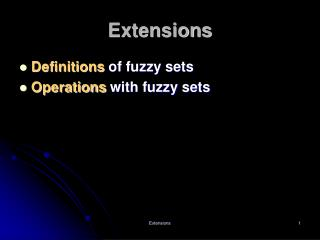# Extensions - PowerPoint PPT PresentationDownload PresentationExtensions

ExtensionsDownload Presentation## Extensions

- - - - - - - - - - - - - - - - - - - - - - - - - - - E N D - - - - - - - - - - - - - - - - - - - - - - - - - - -
##### Presentation Transcript

1. Extensions • Definitions of fuzzy sets • Operations with fuzzy sets Extensions

2. Types of fuzzy sets • Interval-valued fuzzy set • Type two fuzzy set • Type m fuzzy set • L-fuzzy set Extensions

3. Interval-value fuzzy set1 • A membership function based on the latter approach does not assign to each element of the universal set one real number, but a closed interval of real numbers between the identified lower and upper bounds. where ([0,1]) denotes the family of all closed intervals of real numbers in [0,1]. Extensions

4. Interval-value fuzzy set2 Extensions

5. Type 2 fuzzy set1 • A fuzzy set whose membership values are type 1 fuzzy set on [0,1]. (fuzzy set whose membership function itself is a fuzzy set) Extensions

6. Type 2 fuzzy set2 Extensions

7. Type m fuzzy set • A fuzzy set in X whose membership values are type m-1 (m>1) fuzzy sets on [0,1]. Extensions

8. L-fuzzy set • The membership function of an L-fuzzy set maps into a partially ordered set, L. Extensions

9. 集合之二元關係 • 給定集合A及集合B，直積AB的每個子集R都叫從A到B的關係，當(a,b)R時，稱a,b適合關係R，記作aRb。 • 設非空的R是集合A上的二元關係 • 每個aA都有aRa，則稱其具備自反性。 • 若由aRb必可推出bRa，則稱其具備對稱性。 • 若由aRb及bRa必可推出a=b，則稱其具備反對稱性。 • 若由aRb及bRc必可推出aRc，則稱其具備傳遞性。 Extensions

10. 偏序(partially ordered)集 • 集合上具備自反性、反對稱性及傳遞性的關係叫做偏序關係。 • 具偏序關係之集合稱為偏序集。 Extensions

11. Lattice (格) • 若非空之偏序集L的任何個元素構成的集合，既有上確界也有下確界，則偏序集(L，≤)叫做格。 Extensions

12. 格的主要性質1 • 設(L，≤)是格如果在L上規定二元運算∨及∧，a∨b=sup{a，b} ，a∧b=inf{a，b} ，則此二元運算滿足如下算律。 • 冪等律 • a∨a=a • a∧a=a Extensions

13. 格的主要性質2 • 交換律 • a∨b=b∨a • a∧b=b∧a • 結合律 • (a∨b)∨c=a∨(b∨c) • (a∧b) ∧c=a∧(b∧c) • 吸收律 • a∧(a∨b)=a • a∨(a∧b)=a Extensions

14. Operations on fuzzy sets • Fuzzy complement • Fuzzy intersection (t-norms) • Fuzzy union (t-conorms) • Aggregation operations Extensions

15. Fuzzy complements1 C:[0,1]→[0,1] To produce meaningful fuzzy complements, function c must satisfy at least the following two axiomatic requirements: (axiomatic skeleton for fuzzy complements) • Axiom c1. • c(0)=1 and c(1)=0 (boundary conditions)(邊界條件) • Axiom c2. • For all a,b  [0.1], if a≤b, then c(a)≥c(b) (monotonicity)(單調) Extensions

16. Fuzzy complements2 Two of the most desirable requirements: • Axiom c3. • c is a continuous function.(連續) • Axiom c4. • c is involutive, which means that c(c(a))=a for each a  [0,1](復原) Extensions

17. Satisfy c1, c2 Satisfy c1,c2,c3 Satisfy c1~c4 Fuzzy complements3Example Extensions

18. Equilibrium of a fuzzy complement c • Any value a for which c(a)=a • Theorem 1: Every fuzzy complement has at most one equilibrium • Theorem 2: Assume that a given fuzzy complement c has an equilibrium ec, which by theorem 1 is unique. Then a≤c(a) iff a≤ec and a≥c(a) iff a≥ec • Theorem 3: If c is a continuous fuzzy complement, then c has a unique equilibrium. Extensions

19. Fuzzy union (t-conorms) / Intersection (t-norms) • Union u:[0,1][0,1]→[0,1] • Intersection i:[0,1][0,1]→[0,1] Extensions

20. Axiomatic skeleton(t-conorms / t-norms)1 • Axiom u1/i1 (Boundary conditions) • u(0,0)=0; u(0,1)=u(1,0)=u(1,1)=1 • i(1,1)=1; i(0,1)=i(1,0)=i(0,0)=0 • Axiom u2/i2 (Commutative) • u(a,b)=u(b,a) • i(a,b)=i(b,a) Extensions

21. Axiomatic skeleton(t-conorms / t-norms)2 • Axiom u3/i3 (monotonic) If a≤a’, b≤b’ • U(a,b) ≤u(a’,b’) • i(a,b) ≤i(a’,b’) • Axiom u4/i4 (associative) • u(u(a,b),c)=u(a,u(b,c)) • i(i(a,b),c)=i(a,i(b,c)) Extensions

22. Additional requirements(t-conorms / t-norms)1 • Axiom u5/i5 (continuous) • u/i is a continuous function • Axiom u6/i6 (idempotent)(冪等) • u(a,a)=i(a,a)=a Extensions

23. Additional requirements(t-conorms / t-norms)2 • Axiom u7/i7 (subidempotency) • u(a,a)>a • i(a,a)<a • Axiom u8/i8 (strict monotonicity) • a1<a2 and b1<b2 implies u(a1,b1)<u(a2,b2) • a1<a2 and b1<b2 implies i(a1,b1)<i(a2,b2) • Theorem 4 • The standard fuzzy union/intersection is the onlyidempotent and continuous t-conorm/t-norm (i.e., the only function that satisfies Axiom u1/i1~u6/i6) Extensions

24. Cartesian Product of fuzzy set • mth power of a fuzzy set Some t-conorms/t-norms2 Extensions

25. Algebraic sum where Some t-conorms/t-norms3 Extensions

26. Bounded sum where Some t-conorms/t-norms4 Extensions

27. Bounded difference where Some t-conorms/t-norms5 Extensions

28. Algebraic product where Some t-conorms/t-norms6 Extensions

29. Example • Let Extensions

30. Some theorem of t-conorms/t-norms • Theorem 5 • For all a,b [0,1], u(a,b) ≥max(a,b) • Theorem 6 • For all a,b [0,1], u(a,b) ≤umax(a,b) • Theorem 7 • For all a,b [0,1], i(a,b) ≤min(a,b) • Theorem 8 • For all a,b [0,1], i(a,b) ≥imin(a,b) Extensions

31. Aggregation operations • Definition • Operations by which several fuzzy sets are combined to produce a single set. h:[0,1]n→[0,1] for n≥2 Extensions

32. Axiomatic requirements • Axiom h1 • h(0,0,…,0)=0 and h(1,1,…,1)=1 (boundary conditions) • Axiom h2 • For any pair (ai,bi), ai,bi[0,1] if ai≥bi i, then h(ai) ≥h(bi) (monotonic increasing) • Axiom h3 • h is a continuous function. Extensions

33. Additional axiomatic requirements • Axiom h4 • h is a symmetric function in all its arguments; that is, h(a1,a2,…,an)=h(ap(1),ap(2),…,ap(n)) for any permutation p on Nn. • Axiom h5 • H is an idempotent function; that is, h(a,a,…,a)=a Extensions

34. Averaging operations • Any aggregation operation h that satisfies Axioms h2 and h5 satisfies also the inequalities: • min(a1,a2,…,an)≤h(a1,a2,…,an) ≤max(a1,a2,…,an) • All aggregations between the standard fuzzy intersection and the standard fuzzy union are idempotent. • These aggregation operations are usually called averaging operations. Extensions

35. Example for averaging operation • Generalized means (satisfies h1 through h4) α:parameter, αR (α≠0) Extensions

36. Criteria for selecting appropriate aggregation operators • Axiomatic strength • Empirical fit • Adaptability • Numerical efficiency • Compensation • Range of compensation • Aggregating behavior • Required scale level of membership functions Extensions